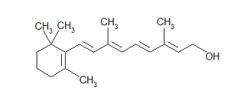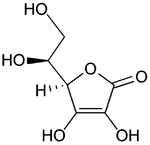• Shuffle
Toggle On
Toggle Off
• Alphabetize
Toggle On
Toggle Off
• Front First
Toggle On
Toggle Off
• Both Sides
Toggle On
Toggle Off
Toggle On
Toggle Off
Front

## Card Range To Study

throughPlay buttonPlay buttonProgress

1/48

Click to flip

Use LEFT and RIGHT arrow keys to navigate between flashcards;

Use UP and DOWN arrow keys to flip the card;

H to show hint;

 Compare the magnitude of the forces of the solvent and solute particles in a solution. The forces of attraction between the solvent and solute particles should be greater or equal in magnitude to the forces between solute-solute particles or solvent-solvent particles to ultimately form a solution. During the formation of a solution, three general energy processes occur. List these processes and list if they are endothermic or exothermic. 1. Separating solute molecules (endothermic) 2. Separating solvent molecules (endothermic) 3. Attractive forces between solvent and solute molecules (exothermic) True or false: Usually when solutions are spontaneously formed, they use energy. False. Spontaneous solution formation is usually exothermic. What is the difference between solute and solvent ? Solvent is usually the substance present in a larger amount than solute. Why does increasing the entropy of a system usually make the formation of the solution spontaneous ? Because by doing so the overall energy of the system is lowered. True or false: Dissolution is a chemical change. False. It is a physical change. Define a saturated solution. This is a solution that holds as much dissolved solute as possible at a specific temperature. Define an unsaturated solution. A solution where less than the maximum amount of solute is dissolved. Define a supersaturated solution. A solution that holds more solute than normally possible at a specific temperature. True or false: The more similar the inter molecular reactions between a solute and solvent, the more likely they will form a solution. True. What is the term for fluids that will mix with each other in all proportions ? Miscible. True or false: Hydrocarbons are polar molecules. False. Hydrocarbons are non-polar molecules.Identify this compound. Vitamin A. Why is Vitamin A not soluble in water ? Because it is a non-polar molecule.Identify this compound. Vitamin C. Why would vitamin C be soluble in water ? Because it is a polar compound. Which of these substances would dissolve either in CCl4 or water ? 1. C7H16 2. Na2SO4 3. HCl 4. I2 1. CCl4 2. Water 3. Water 4. CCL4 What is the correlation between mass of a gas particle and its solubility in water ? The heavier the gas particle, the more soluble. What is the effect of pressure on gas solubility ? Increasing the pressure of a gas in a container with water will increase its solubility. Give Henry's law of gas solubility. S = Pk. What is the effect of temperature on solid solubility ? With an increase in temperature, the solubility of a gas also increases. What is the effect on solubility of Ce2(SO4)3 with an increase in temperature ? It becomes less soluble with an increase in temperature. What is the effect on gas solubility with an increase in temperature ? An increase in temperature causes the gas to become less soluble. Give the equation for expressing concentration by using- Mass Percentage. [(mass of A in solution)/(total mass of solution)] x 100. Give the equation for expressing concentration by using- Mole Fraction. (moles of component A)/(total moles of all components) Give the equation for expressing concentration by using- Molarity. (moles of solute)/(liters of solution). True or false: Molarity changes with a change in temperature. True. Because volume affects molarity and temperature affects volume we will see a change in molarity if the temperature changes. Give the equation for expressing concentration by using- Molality. (moles of solute)/(kg of solvent). True or false: Molarity changes with a change in temperature. False. Molality is only dependent on mass and moles, these variable do not change with a change in temperature. Which equation will you use to 'link' molality and molarity ? Density. An experiment calls for 36.0 g of a 5.00% aqueous solution of potassium bromide. Describe how you would make up such a solution. We make the solution by mixing 1.8 g KBr in 34.2 g water. Calculate the molarity of a solution of 0.0678g of NaCl in 25ml of total solution. 0.0464 M. Iodine dissolves in a variety of organic solvents. For example, in methylene chloride, it forms an orange solution. What is the molality of a solution of 5.00 g iodine, I2, in 30.0 g of methylene chloride, CH2Cl2? 0.657 m. A solution of iodine, I2, in methylene chloride, CH2Cl2, contains 5.00 g I2 and 56.0 g CH2Cl2. What is the mole fraction of each component in this solution? X(I2) = 0.0290 X(CH2Cl2) = 0.971 A bottle of bourbon is labeled 94 proof, meaning that it is 47% by volume of alcohol in water. What is the mole fraction of ethyl alcohol, C2H5OH, in the bourbon? The density of ethyl alcohol is 0.80 g/mL. X(ethanol) = 0.22 A 3.6 m solution of calcium chloride, CaCl2, is used in tractor tires to give them weight. The addition of CaCl2 also prevents water in the tires from freezing at temperatures above –20°C. What are the mole fractions of CaCl2 and water in such a solution? X(CaCl2) = 0.061 X(H2O) = 0.94 A solution contains 8.89 × 10-3 mole fraction of I2 dissolved in 0.9911 mole fraction of CH2Cl2 (methylene chloride). What is the molality of I2 in this solution? 0.106 m. Citric acid, HC6H7O7, is often used in fruit beverages to add tartness. An aqueous solution of citric acid is 2.331 m HC6H7O7. What is the molarity of the solution? The density of the solution is 1.1346 g/mL. 1.827 M. What is the single factor that influences a solution's colligative properties ? The number of solute particles present. Name the four colligative properties. 1. Vapour pressure lowering 2. Boiling point elevation 3. Freezing point depression 4. Osmotic pressure Describe why the vapour pressure is lowered when a non-volatile solute is dissolved in a volatile solvent. The non-volatile solute makes it harder for the volatile molecules to escape the solution to enter the gas phase. This results in a lower number of molecules in the gas phase and thus the pressure is lowered. Give the equation of Raoult's law. Pa = Xa x Pa. Which variable is proportional to the increase in boiling point or decrease in freezing point of a solution ? Molality. Give the equation for osmotic pressure. Pi = MRT. Describe what will happen to a blood cell when the plasma is hypertonic. The cell will result in crenation, water flows out of the cell. Descrribe what will happen to a blood cell when the plasma is hypotonic. The cell will result in haemolysis, water will flow into the cell. What is a colloid ? A particle that is larger than an individual atom or molecule, but too small to be settled by gravity. What is the phenomenon called where colloids scatter rays of light ? The Tyndall effect.